Home > INT3 > Chapter 7 > Lesson 7.2.5 > Problem7-113

7-113.
1. Solve each of the following equations. Be sure to check your solutions. Homework Help ✎

1. 3 +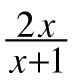= 5x

2. x2 + 6x + 9 = 2x2 + 3x + 5

3. 8 –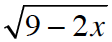= x + 3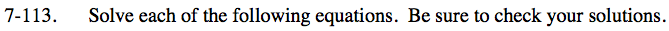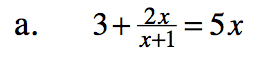To remove the denominator, multiply both sides of (or each term in) the equation by (x + 1).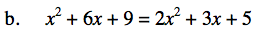x = 4, −1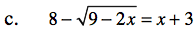Subtract 8 from both sides and divide both sides by −1.

$\sqrt{9-2x}=5-x$

After setting the resulting equation equal to zero and solving the quatdratic equation, x = 4.

Square both sides and simplify.

$\left(\sqrt{9-2x}\right)^{2}=(5-x)^2$

9 − 2x = x2 − 10x + 25